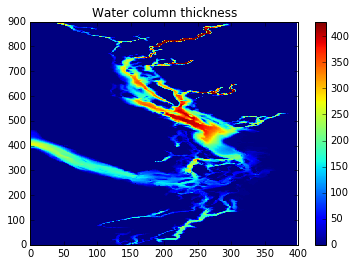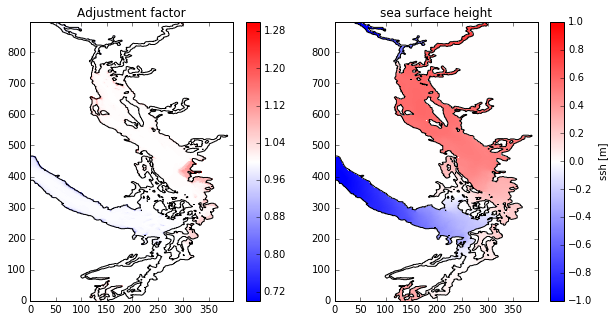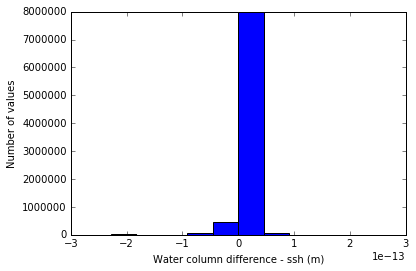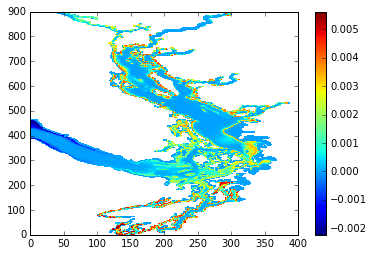This notebook is used to develop routines for building the time-dependent scale factors and depths in NEMO with variable volume (vvl).

In :
import netCDF4 as nc
import numpy as np
import matplotlib.pyplot as plt

from salishsea_tools import viz_tools, grid_tools

%matplotlib inline

The vertical grid spacing in NEMO with variable volume is time-dependent. NEMO dsitributes the expanssion/compression of the water column equally across all water cells according the this formula:

$e3t_t = e3t_0(1 + \eta / H)$

where $e3t_t$ is the time dependent vertical grid spacing, $\eta$ is the sea surface height and $H$ is the water column depth. $e3t_0$ is the initial vertical grid spacing which is stored in the mesh mask file. It is the grid spacing if the sea surface heigh were zero. Also, $H = \sum_{k=0}^{jpk} e3t_0 *tmask$ where tmask is the mask on the T-grid and $jpk$ is the last grid cell.

The grid spacing on the w grid also follow this simple formula, as well as the depths.

$e3w_t = e3w_0(1 + \eta / H)$

$gdept_t = gdept_0(1 + \eta / H)$

$gdepw_t = gdepw_0(1 + \eta / H)$

Where $e3w$ means vertical grid spacing on W grid, $gdept$ means depth levels on T grid and $gdepw$ means depth levels on W grid. the underscore 0 indicates initial values from mesh mask file and underscore t means time-dependent. Note that in this formulation gdepw_t(k=0) = 0 always, so these depths can be thtough of as a pressure. To find the depths relative to $\eta=0$ you just need to subtract the sea surface height. See this notebook:

The U/V grids are slighlty more complicated and will be considered later. Basically, the ssh has to be interpolated onto the U/V grids.

Functions:

1. Calculate the water column thickness
2. Calculate adjustment factor, $adj = 1 + \eta / H 3. Calculate time-dependent grids Loading Data¶ In : mesh_mask = nc.Dataset('/data/nsoontie/MEOPAR/NEMO-forcing/grid/mesh_mask_SalishSea2.nc') grid = nc.Dataset('/data/nsoontie/MEOPAR/NEMO-forcing/grid/bathy_meter_SalishSea2.nc') bathy = grid.variables['Bathymetry'][:] # Sample file for testing calculations fT = nc.Dataset('/results/SalishSea/nowcast/01apr15/SalishSea_1h_20150401_20150401_grid_T.nc') In : ssh = fT.variables['sossheig'] tmask = mesh_mask.variables['tmask'][:] e3t_0 = mesh_mask.variables['e3t'][:] gdept_0 = mesh_mask.variables['gdept'][:] gdepw_0 = mesh_mask.variables['gdepw'][:] e3w_0 = mesh_mask.variables['e3w'][:] Functions¶ Calculating$H$¶ In : def calculate_H(e3t0, tmask): """Calculate the initial water column thickness (H). :arg e3t0: initial vertical scale factors on T-grid. Dimensions: (depth, y, x). :type e3t0: :py:class:numpy.ndarray :arg tmask: T-grid mask. Dimensions: (depth, y, x) :type tmask: :py:class:numpy.ndarray :returns: the initial water column thickness. Dimensions: (y, x) """ H = np.sum(e3t0*tmask, axis=0) return H Check¶ Timing In : % timeit H = calculate_H(e3t_0[0,...], tmask[0,...]) 10 loops, best of 3: 38.9 ms per loop Plot$H\$.

In :
H = grid_tools.calculate_H(e3t_0[0,...], tmask[0,...])
print(H.shape)
plt.pcolormesh(H)
plt.colorbar()
plt.title('Water column thickness')
(898, 398)
Out:
<matplotlib.text.Text at 0x7f23aedcba90>In :
"""Calculate the time-dependent adjustment factor for variable volume in
NEMO. adj = (1+ssh/H) and e3t_t = e3t_0*adj

:arg H:  Water column thicnkess. Dimension: (y, x)
:type H: :py:class:numpy.array

:arg ssh: the model sea surface height. Dimensions: (time, y, x)
:type ssh: :py:class:numpy.ndarray

:returns: the adjustment factor with dimensions (time, y, x)
"""
with np.errstate(divide='ignore', invalid='ignore'):
one_over_H = 1 / H
one_over_H = np.nan_to_num(one_over_H)
adj = (1 + ssh * one_over_H)

Check¶

Timing

In :
1 loop, best of 3: 111 ms per loop
In :
(24, 898, 398)

Adjustment factor should be >1 when ssh >0. Quickly visualize.

In :
t=0
fig,axs=plt.subplots(1,2,figsize=(10,5))
ax=axs
vmin=.7,vmax=1.3,cmap='bwr')
plt.colorbar(mesh, ax=ax)
viz_tools.plot_coastline(ax,grid)
ax=axs
mesh=plt.pcolormesh(ssh[t,:,:], vmin=-1,vmax=1,cmap='bwr')
cbar = plt.colorbar(mesh, ax=ax)
cbar.set_label('ssh [m]')
viz_tools.plot_coastline(ax,grid)
ax.set_title('sea surface height')
Out:
<matplotlib.text.Text at 0x7f23ae631d68>In :
# Check adjustment factor is >=1 where ssh >=0.
inds=np.where(ssh[:]>=0)
Out:
True
In :
# Check adjustment factor is <1 where ssh <0.
inds=np.where(ssh[:]<0)
Out:
True
In :
# Check adj <=1 is false when ssh >=0
inds=np.where(ssh[:]>=0)
Out:
False
In :
# Check adj >=1 is false when ssh <0
inds=np.where(ssh[:]<0)
Out:
False

Calculating scale factors and depths¶

In :
def calculate_time_dependent_grid(
e3t0,
ssh,
input_vars,
):
""" Calculate the time dependent vertical grids and scale factors for
variable volume in NEMO.

:arg e3t0: initial vertical scale factors on T-grid.
Dimensions: (depth, y, x).
:type e3t0: :py:class:numpy.ndarray

:arg tmask: T-grid mask. Dimensions: (depth, y, x)
:type tmask: :py:class:numpy.ndarray

:arg ssh: the model sea surface height. Dimensions: (time, y, x)
:type ssh: :py:class:numpy.ndarray

:arg input_vars: A dictionary of the initial grids/scale factors to be
translated into time_dependent. Example keys can be
'e3t_0', 'gdept_0', 'e3w_0', 'gdepw_0'. A dictionary with
correspinding time-dependent grids is returned, where the
keys are now 'e3t_t', 'gdept_t', 'e3w_0', 'gdepw_0'.
:typ input_vars: dictionary
:type return_vars: list of strings

:returns: A dictionary containing the desired time dependent vertical
scale factors on t and w grids and depths on t and w grids.
Dimensions of each: (time, depth, y, x)
"""
H = calculate_H(e3t0, tmask)
adj = np.expand_dims(adj, axis=1)  # expand to give depth dimension
# Time-dependent grids
return_vars = {}
for key in input_vars:
return_key = '{}t'.format(key[0:-1])
return_vars[return_key] = input_vars[key] * adj

return return_vars

Check¶

Timing

In :
input_vars = {'e3t_0': e3t_0,
'e3w_0': e3w_0,
'gdept_0': gdept_0,
'gdepw_0': gdepw_0}
In :
% timeit grids = calculate_time_dependent_grid(e3t_0[0,...], tmask[0,...], ssh, input_vars)
1 loop, best of 3: 2.5 s per loop
In :
grids = calculate_time_dependent_grid(e3t_0[0,...], tmask[0,...], ssh, input_vars)
In :
e3t_t = grids['e3t_t']
e3w_t = grids['e3w_t']
gdept_t = grids['gdept_t']
gdepw_t = grids['gdepw_t']

1. Water column thickness¶

Calculate total water column by summing vertical scale factors for both initial and time dependent scale factors. In the time-dependent case, the thickness should shange with the sea surace height.

In :
H_0 = np.sum(e3t_0*tmask, axis=1) # initial water column thickness
H_t = np.sum(e3t_t*tmask, axis=1) # time-dependent water column thickness
In :
#difference in water column thickness - should be explained by the ssh
diff_depth = H_t - H_0
diff_ssh = diff_depth-ssh
In :
print('Mean difference with ssh: {} m'.format(diff_ssh.mean()))
print('Max difference with ssh: {} m'.format(diff_ssh.max()))
print('Min difference with ssh: {} m'.format(diff_ssh.min()))
Mean difference with ssh: -2.8955083512058694e-16 m
Max difference with ssh: 2.2737367544323206e-13 m
Min difference with ssh: -2.2737367544323206e-13 m
In :
plt.hist(diff_ssh.flatten())
plt.xlabel('Water column difference - ssh (m)')
plt.ylabel('Number of values')
Out:
<matplotlib.text.Text at 0x7f23ae50d240>Ok, so the water column is expanding and compressing by exactly the ssh (to within reasonable error). That makes sense.

2. Depth of bottom ocean point¶

Next, check to make sure the bottom hasn't moved! That is, the w point that represents the bathymetry shouldn't be affected.

Note the depth variables are like a pressure, that is, gdepw(k=0) = 0 because it is at the surface. So the bottom feels the ssh moving up and down. So, compare gdepw_t[bottom] - ssh to gdepw_0[bottom].

In :
bottom_0 = np.zeros(H_0[0,:,:].shape)
bottom_t = np.zeros(H_t.shape)
for i in np.arange(H_0[0,:,:].shape):
for j in np.arange(H_0[0,:,:].shape):
level = mbathy[j,i]
bottom_0[j,i] = gdepw_0[0,level,j,i]
bottom_t[:,j,i] = gdepw_t[:,level,j,i]

At a single point.

In :
j=400
i=300
diff = bottom_t - bottom_0 - ssh
In :
bottom_0[j,i]
Out:
23.375
In :
bottom_t[:,j,i]
Out:
array([ 23.77571649,  23.44446205,  23.0530772 ,  22.68543079,
22.46104562,  22.43946222,  22.631597  ,  22.99489061,
23.45437265,  23.92271361,  24.28867064,  24.47369503,
24.42435875,  24.16847472,  23.79394418,  23.3641584 ,
22.97786956,  22.74423954,  22.7199077 ,  22.89045275,
23.17842863,  23.5164112 ,  23.81913159,  23.97568495])
In :
diff[:,j,i]
Out:
array([  8.69166276e-04,   1.50665308e-04,  -6.98260355e-04,
-1.49569661e-03,  -1.98239487e-03,  -2.02920993e-03,
-1.61246373e-03,  -8.24468832e-04,   1.72161701e-04,
1.18800748e-03,   1.98177944e-03,   2.38310297e-03,
2.27609108e-03,   1.72107082e-03,   9.08702680e-04,
-2.35157594e-05,  -8.61388016e-04,  -1.36813865e-03,
-1.42091514e-03,  -1.05099773e-03,  -4.26369280e-04,
3.06725197e-04,   9.63334934e-04,   1.30290394e-03])

Over the whole domain, at a single time.

In :
t=10
plt.colorbar()
Out:
<matplotlib.colorbar.Colorbar at 0x7f23ae3c99b0>In :
print(np.max(diff))
print(np.min(diff))
print(np.mean(diff))
0.00629676075017
-0.00619416041651
-5.35265373469e-06

Next¶

• Write this functions into a module grid_tools.py in salishsea_tools
• Figure out U/V grids
In [ ]: